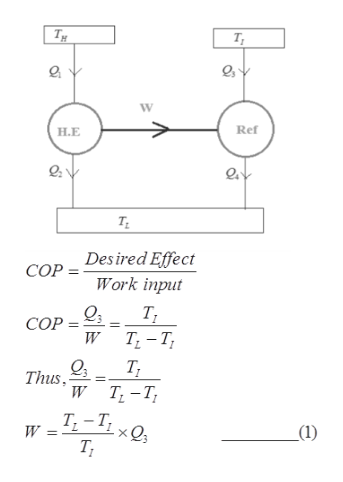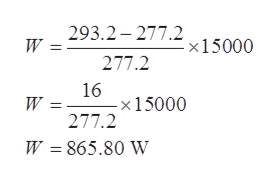# Consider three reservoirs that are TH = 473.2 K, TL = 293.2 K and TI = 277.2 K.  A Carnot heat engine can be reversed and used as either a heat pump or a refrigerator.  In the case of a heat pump the heat flow to the higher temperature reservoir is the desired output and it is used to heat a room or building.  In the case of the refrigerator the desired energy flow is the heat in from a region to be cooled.  For the heat pump or refrigerator, the work input is provided by electricity.A region is to be cooled to a temperature of 277 K using a heat powered Carnot refrigerator.  The heat that must be removed is 15,000 W.  The heat rejected from the refrigerator is to a heat reservoir at TL. The heat input to this device is from a reservoir  at TH.  This is a Carnot model of an absorber refrigeration system.  In it a Carnot heat engine operates between reservoirs at TH and TL.  The work output from this Carnot heat engine is the input to a Carnot refrigerator that operates between TI and TL. Consider the cooling for a period of 3 hours. Answer the following:a)Determine the heat energy input to this Carnot refrigerator.b) The cost of the fuel to operate this system is \$0.075/kWh.  What is the cost to cool the room for 3 hours?

Question
120 views

Consider three reservoirs that are TH = 473.2 K, T= 293.2 K and TI = 277.2 K.  A Carnot heat engine can be reversed and used as either a heat pump or a refrigerator.  In the case of a heat pump the heat flow to the higher temperature reservoir is the desired output and it is used to heat a room or building.  In the case of the refrigerator the desired energy flow is the heat in from a region to be cooled.  For the heat pump or refrigerator, the work input is provided by electricity.

A region is to be cooled to a temperature of 277 K using a heat powered Carnot refrigerator.  The heat that must be removed is 15,000 W.  The heat rejected from the refrigerator is to a heat reservoir at TL. The heat input to this device is from a reservoir  at TH.  This is a Carnot model of an absorber refrigeration system.  In it a Carnot heat engine operates between reservoirs at TH and TL.  The work output from this Carnot heat engine is the input to a Carnot refrigerator that operates between TI and TL. Consider the cooling for a period of 3 hours.

a)Determine the heat energy input to this Carnot refrigerator.

b) The cost of the fuel to operate this system is \$0.075/kWh.  What is the cost to cool the room for 3 hours?

check_circle

Step 1

Given data:

The temperature of three reservoir are:

TH = 473.2 K

TL = 293.2 K

TI = 277.2 K

Heat removed Q = 15,000 W

Step 2

(a); The Carnot refrigerator that operates between TI and TL the equation for coefficient of performance is given as:help_outlineImage TranscriptioncloseTy T Ref Н.Е TL Desired Effect СОР Work input СОР Т, -Т, W Thus W Т, -Т, W _(1) т, fullscreen
Step 3

Put the values in e...help_outlineImage Transcriptionclose293.2 277.2 W = x15000 277.2 16 -x1 5000 277.2 W = W 865.80 W fullscreen

### Want to see the full answer?

See Solution

#### Want to see this answer and more?

Solutions are written by subject experts who are available 24/7. Questions are typically answered within 1 hour.*

See Solution
*Response times may vary by subject and question.
Tagged in

### Mechanical Engineering